# Hypo-Dirichlet algebra

Jump to: navigation, search

Letbe a uniform algebra onandthe algebra of all continuous functions on(cf. also Algebra of functions). The algebrais called a hypo-Dirichlet algebra if the closure of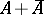has finite codimension in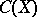, and the linear span of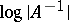is dense in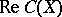, whereis the family of invertible elements of. Hypo-Dirichlet algebras were introduced by J. Wermer [a4].

Letbe the boundary of a compact subsetin the complex plane whose complement has only finitely many components. Let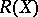be the algebra of all functions onthat can be uniformly approximated by rational functions with poles off(cf. also Padé approximation; Approximation of functions of a complex variable). Thenis a hypo-Dirichlet algebra [a3].

Letbe a hypo-Dirichlet algebra onanda non-zero complex homomorphism of. Ifis a representing measure onsuch thatforin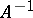, thenis unique. For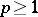, the abstract Hardy space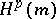is defined as the closure ofin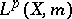(cf. also Hardy spaces). Then a lot of theorems for the concrete Hardy space defined byare valid for abstract Hardy spaces [a2]. Using such a theory, J. Wermer [a4] showed that if the Gleason part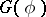ofis non-trivial (cf. also Algebra of functions), thenhas an analytic structure.

See also Dirichlet algebra.